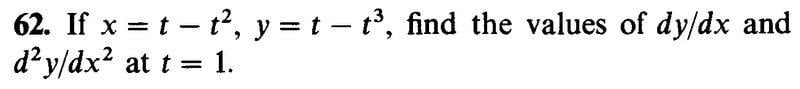# Second derivative in parametric equations

## Homework StatementOnly the second part

## Homework Equations

Second derivative:
$$\frac{d^2y}{dx^2}=\frac{d}{dx}\frac{dy}{dx}$$

## The Attempt at a Solution

$$dx=(1-2t)\,dt,~~dy=(1-3t^2)\,dt$$
Do i differentiate the differential dt?
$$d^2x=(-2)\,dt^2,~~d^2y=(-6)t\,dt^2$$
$$\frac{d^2y}{dx^2}=\frac{d^2y/dt^2}{d^2x/dt^2}=\frac{-6t}{-2}=...=3$$
The answer should be -2

## Answers and Replies

Orodruin
Staff Emeritus
Science Advisor
Homework Helper
Gold Member
You cannot do it like that. Use the chain and Leibniz rules.

Ray Vickson
Science Advisor
Homework Helper
Dearly Missed

## Homework Statement

View attachment 210022
Only the second part

## Homework Equations

Second derivative:
$$\frac{d^2y}{dx^2}=\frac{d}{dx}\frac{dy}{dx}$$

## The Attempt at a Solution

$$dx=(1-2t)\,dt,~~dy=(1-3t^2)\,dt$$
Do i differentiate the differential dt?
$$d^2x=(-2)\,dt^2,~~d^2y=(-6)t\,dt^2$$
$$\frac{d^2y}{dx^2}=\frac{d^2y/dt^2}{d^2x/dt^2}=\frac{-6t}{-2}=...=3$$
The answer should be -2
Set ##y' = dy/dx = y'(t)## and then comput ##d^2 y/dx^2 = dy'/dx## paremetrically

$$\frac{d^2y}{dx^2}=\frac{d}{dx}\frac{dy}{dx}=\frac{d}{dx}\left( \frac{1-3t^2}{1-2t} \right)$$
$$=\frac{d \left( \frac{1-3t^2}{1-2t} \right)/dt}{dx/dt}=\frac{d \left( \frac{1-3t^2}{1-2t} \right)/dt}{1-2t}$$
It comes out right, -2
Thank you Orodruin and Ray

•I like Serena